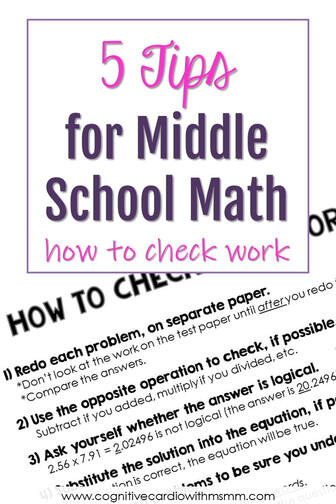# Helping Students Learn How to Check Math Work

## Strategies for Students to Check Their Own Math AnswersWhen my 6th grade math students finish their math assessments and want to hand them in, I always ask, “Did you check your work?”
Often the answer is “yes,” but if it’s not, I won’t take the paper until the student does check their work. So, they go back to the assessment and ‘check’ for a couple minutes and are ready to hand the assessment in again.
How do they do this math checking?

Do you know what I’m going to say?

Here’s what my math students often do (until I teach them math checking strategies) – they look at the math questions, basically make sure that all the questions were answered, and say they’ve checked their work.
Unless they are clearly taught otherwise, many students seem to believe that “checking your math work” means “checking to see if everything is done.”

## Five Strategies for Checking Math Answers

The following strategies for checking math answers are the ones I’ve found to be the most helpful to my math students.
They aren’t always thrilled by the extra time and effort this math checking takes, but they do admit that it helps:-)

1) Redo the math problemson a separate piece of paper, without looking at the work that was already done.

• When students look at the work they already did, it influences them and they sometimes make the same mistake again.

After redoing the math problem, they compare their original math work and answer with the redo. This can take a while, but when students are ready to hand in an assessment with lots of class time left, I recommend they take the time to do this.

2) Use the opposite operation to check, if possible. If the problem was an addition problem, subtract one of the addends from the sum. If it was a division problem, use multiplication to check, and so on.

3) Ask whether the answer is logical.
If the problem was 2.56 x 7.91, an answer of 2.02496 (instead of 20.2496), is not logical.

• This is where their estimating skills come in to play. I take a lot of time in my math instruction to model estimating and how to determine if an answer to a math problem is reasonable.

4) Substitute the solution into the equation, if possible. If the solution is correct, the equation will be true.

5) If the test includes word problems, reread each problem carefully to be sure all of the information was understood correctly.

• Look for numbers that are written as words rather than digits.
• Be sure the answer actually answers the question the problem is asking.

I created a How to Check Your Math Work reminder sheet for students to keep in their notebooks. You can download it if you’d like – there’s a colored version and a black and white version, in case you want to print on colored paper.

What strategies for checking math answers do you teach  students?
How do digital assessments impact the math checking?

## EllieWelcome to Cognitive Cardio Math! I’m Ellie, a wife, mom, grandma, and dog ‘mom,’ and I’ve spent just about my whole life in school! With nearly 30 years in education, I’ve taught:

• All subject areas in 4th and 5th grades
• Math, ELA, and science in 6th grade (middle school)

I’ve been creating resources for teachers since 2012 and have worked in the elearning industry for about five years as well!

If you’re looking for ideas and resources to help you teach math (and a little ELA), I can help you out!